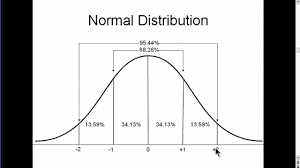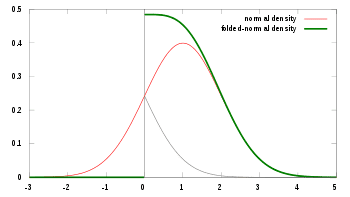# A test for a superluminal neutrino?

• I
RobertGC
TL;DR Summary
An interplanetary test.
The neutrino has been puzzling since its proposal and its experimental confirmation.
There have been experimental anomalies with it for every experiment designed to test it.

One fact that might be quite key is that measurements of the mass-square of the neutrino has consistently shown it to have a negative mass-square:

Why is the square of the neutrino mass negative?
In arXiv:hep-ph/0009291 this is explained by giving the example of:

m2 = -2.5 +- 3.3eV2.

"The negative value of the neutrino mass-square simply means:"

E2/c2 -p2 = m2c2 < 0,

https://physics.stackexchange.com/questions/578556/why-is-the-square-of-the-neutrino-mass-negative

This has led to speculation the neutrino may be a tachyon. Another unusual fact about it is that its cross section gets larger at higher energies and smaller at lower energies. And with a tachyon, in contrast to a bradyon, higher energy means it is going slower, and lower energies means it going faster. This would explain why it would be harder to interact with at the lower energies.

However, with the unfortunate mistake at the OPERA experiment where is was offered a superluminal neutrino to explain an experimental anomaly, any such claim needs to have overwhelming support to confirm it.

I was thinking of having the neutrino travel through the Earth and be detected on the other side. However, even at the speed of light the travel time would be quite short and given the difficulty of detecting the neutrino it would be difficult to get the timing accurate enough to make sure the detection was above background.

Then I wanted a longer travel time. Could we have an accelerator generated neutrino pulse be aimed at the Moon or Mars or Venus, and look for the neutrinos scattered back to be captured here on Earth? Because of the low cross-section it would have to be an extremely dense beam to have enough particles to bounce back to be detectable.

Robert Clark

•weirdoguy and PeroK

Staff Emeritus
This is a misconception built on other misconceptions.

From your last thread, it's clear that - at best - you think we know no more than we did two decades ago. A good starting point might be to catch up before deciding you know how to do neutrino experiments better than the neutrino experimenters.

•russ_watters, vanhees71, ohwilleke and 2 others
Gold Member
2022 Award
Summary:: An interplanetary test.

The neutrino has been puzzling since its proposal and its experimental confirmation.
There have been experimental anomalies with it for every experiment designed to test it.
As @Vanadium 50 pointed out, you REALLY need to do a lot more reading to avoid making incorrect statements..

•vanhees71 and RobertGC
Gold Member
Why is the square of the neutrino mass negative?
In arXiv:hep-ph/0009291 this is explained by giving the example of:

m2 = -2.5 +- 3.3eV2.

"The negative value of the neutrino mass-square simply means:"

E2/c2 -p2 = m2c2 < 0,

https://physics.stackexchange.com/questions/578556/why-is-the-square-of-the-neutrino-mass-negative
Your post is based upon a false premise rooted in a misunderstanding of a somewhat confusing form of notation that does not have the physical implications that you suggest.

The article you cite to doesn't mean what your post suggests that it does.

The result cited is simply a consequence of providing a result in the form of a Gaussian uncertainty (i.e. an uncertainty described in a manner that implicitly assumes that the uncertainty is distributed in a "normal' distribution) in circumstances that are strictly beyond the scope of applicability of Gaussian probability and statistics stated in that form, because we are sure that this isn't the true shape of the probability distribution.

We know that the true probability distribution is almost surely non-Gaussian (i.e. not distributed in the shape of a "normal" probability curve), because the experimental data strongly suggests that the probability distribution of the underlying quantity being measured is heavily concentrated on the lower floor of the probability distribution, even if it is a little bit lazy to do so. The physicists do this because they are trying to shoehorn their results into the format of a normal probability distribution because it is their custom and habit to do so.

If the authors wanted to be really rigorous and mathematically pedantic in their report of their results, they would have reported the probability in a manner consistent with a lower bound of zero for the neutrino mass, because the experimental methods used do not have, and do not purport to have, a way to identify a true imaginary neutrino mass, or superluminal neutrino.

In other words, their experimental result (considered in isolation without joint analysis with other experimental data, and rounding to avoid the impression of spurious accuracy) is consistent with:

* a roughly 74% probability that the neutrino mass is between 0.0 and 0.05 eV/c2 (i.e. the minimum possible mass value that is indistinguishable from zero by this experiment given its limited precision, as distinguished from its accuracy);​
* a roughly 10% probability (i.e. distinguishable from zero with the precision of the experiment but less than one sigma higher than expected) that the neutrino mass is between 0.05 eV/c2 and 0.9 eV/c2;​
* a roughly 13.5% probability (i.e. more than one sigma but less than two sigma higher than expected) that the neutrino mass is between 0.9 eV/c2 and 2.0 eV/c2; and​
* a roughly 2.5% probability (i.e. more than two sigma higher than expected) that the neutrino mass is more than 2.0 eV/c2.​
Obviously, these percentages are rounded. These rough estimates also ignore some small correction factors that imply that the 74% figure is actually slightly low, and the 10% figure is actually slightly high. If I did the mathematics with full rigor, the percentages, rounded to the closest full percentage point, would be closer to 75% and 9%.

These inaccuracies are still smaller than the inaccuracies associated with assuming a Gaussian distribution, rather than fitting the data to a more reasonable probability distribution with a zero bound.

But, ultimately, as long as the trained scientists are aware that their results are being reported in a slightly unrigorous manner so that they don't try to use the results inappropriately in a manner beyond the range of applicability, and so long as everyone reports their results in a manner consistent with this Gaussian error convention, everything works out. Physicists can still make apples to apples comparisons can be made between the results of different experiments, and this isn't a serious problem.

We know from other lines of evidence (mostly cosmology corroborated by neutrino oscillation data and a certain amount of physics intuition) that the true value of the smallest neutrino mass eigenstate is realistically probably less than 0.012 eV/c2, and quite likely less than 0.001 eV/c2, which is a value indistinguishable from zero in the experimental results cited.

So, it makes sense that the probability distribution of possible neutrino masses consistent with the experimental result should be concentrated near the floor of that distribution. Indeed, this result is just what we would expect for a reality as we think it is likely to be, reported in this somewhat quirky method preferred by physicists.

But, a concentration of probabilities near a lower bound in an experiment like this one would imply, contrary to the implied statement made by citing the result in this fashion, that the distribution of uncertainty in probability given the experimental data is not distributions in a Gaussian "normal" distribution, and is instead concentrated at the lower tail of the distribution. So, taken overly literally, the results would suggest and absurd result which is inconsistent with what the experiment actually determined.

If the probability distribution of the neutrino mass that the experimental results suggest were Gaussian, it would look like:But, in fact, the neutrino mass probability distribution implied by these experimental results has a very high peak near zero and goes steadily downward towards the right as the neutrino mass values implied by the experiment get larger.

The real probability distribution of the neutrino mass implied by this experiment looks closer to the green line in the image below, only even more steeply peaked near zero (with the red line peaking at a z= -0.65 value on the z score normalized image below):Notwithstanding this contradiction, it is customary for physicists to refrain from using that level of rigor, and to instead express their experimentally results in the somewhat inartful manner quoted in your original post in this thread.

Rather than having any profound meaning, it is simply a matter of a somewhat sloppy notation convention that the physicists have adopted on a widespread basis, because it is convenient and saves a lot of tedious rote statistical analysis that adds little of substance of the basic conclusion.

This convention of using a Gaussian error format to report values to which a continuous Gaussian probability distribution doesn't apply is widespread in physics, both in other aspects of neutrino physics and in other subfields of physics.

Another Example Of This Form Of Mathematically Non-Rigorous Gaussian Notation

Similarly, for example, the experimental evidence regarding the number of active Standard Model neutrinos is quoted by the Particle Data Group as 2.996 ± 0.007, even though the quantity described is inherently defined such that it must be either zero or a positive integer.

This simply means that the best fit value of the number of active Standard Model neutrino types to the experimental results is exactly three, that experimental result is closer to exactly three than a researcher would expect from random chance, although not dramatically so, that the probability that there are two or fewer such types is more than 142 standard deviations away from the best fit value if the experimental uncertainty has been accurately approximated, and that the probability that there are four or more such types is more than 143 standard deviations away from the best fit value if the experimental uncertainty has been accurately approximated.

Thus, the experimental results is consistent with the theoretically predicted value and may overstate the magnitude of the systemic error in the experiment somewhat, and the probability that the experimental results observed could be other than exactly three consistent with the experimental data collected is so negligibly small that it is essentially indistinguishable from zero.

Last edited:
•russ_watters and vanhees71
Mentor
The first post is so full of misconceptions and wrong statements that this thread doesn't have a chance to go anywhere useful.

•